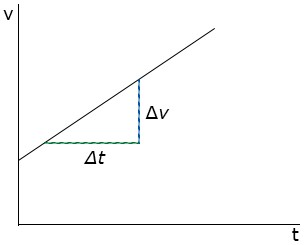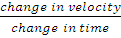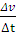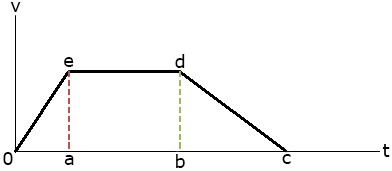### Speed, Velocity and acceleration

Physics SSS2 First Term

Sub Theme: Interaction of matter, space and time

WEEK 3

Speed, Velocity and acceleration

Performance Objectives

Students should be able to;

1. Define speed, velocity and acceleration
2. Show that speed is a scalar quantity while velocity and acceleration are vectors
3. Show on a (V – t) graph the motion of a body
4. Deduce the distance covered between any time intervals on the graph in 3 above

Velocity – Time v-t Graph

Content

1. Gradient of a v-t graph = accelerationAcceleration = gradient = change in velocitychange in timeacceleration(a) =2. Area under a v - t graph = distance.1. Total distance covered.... View More